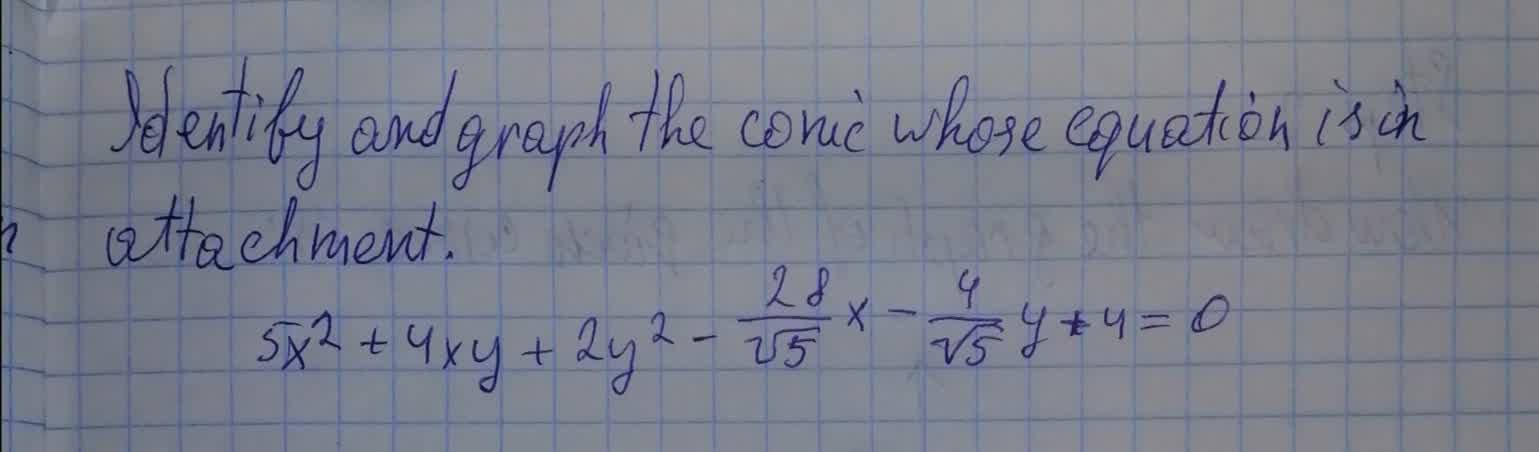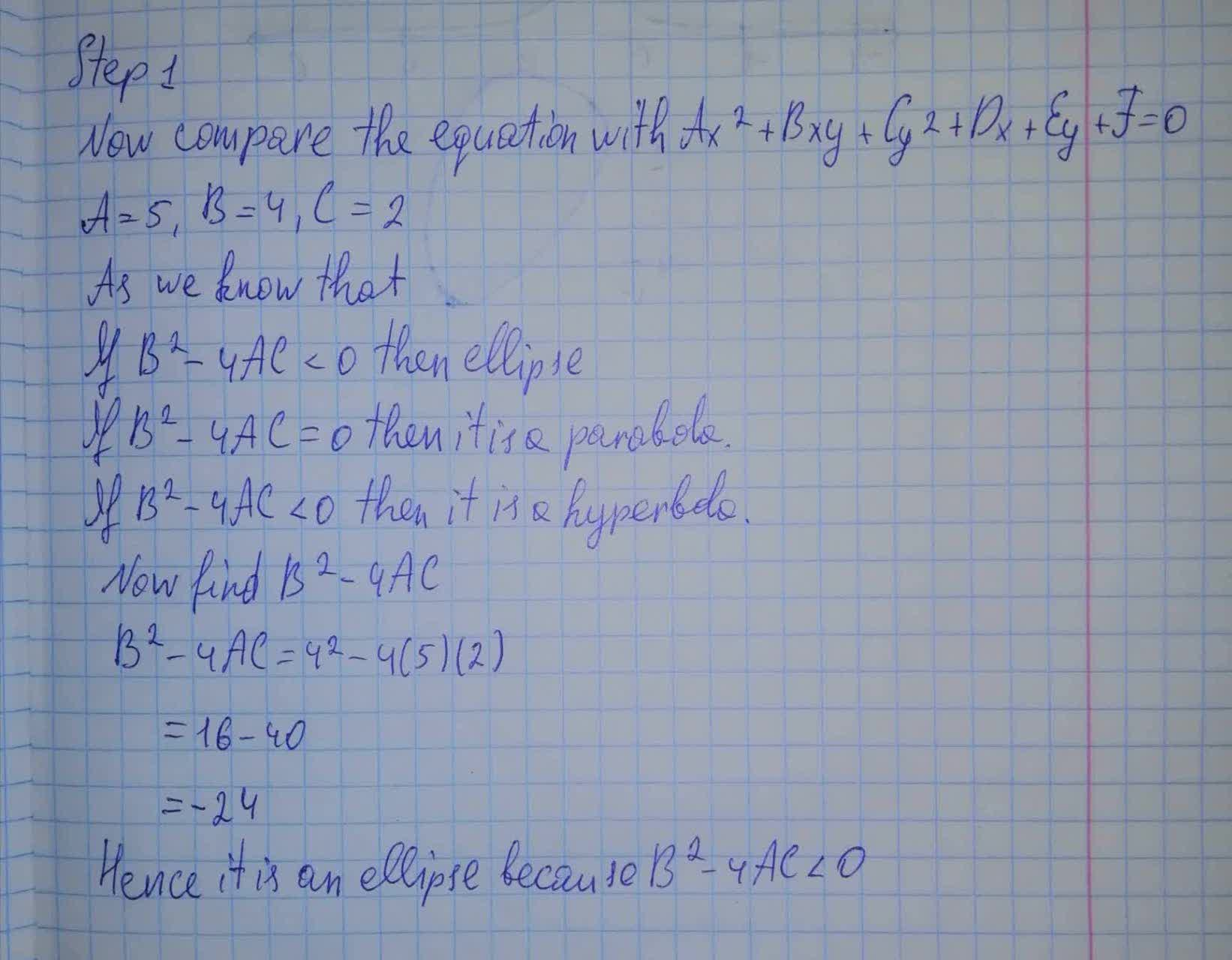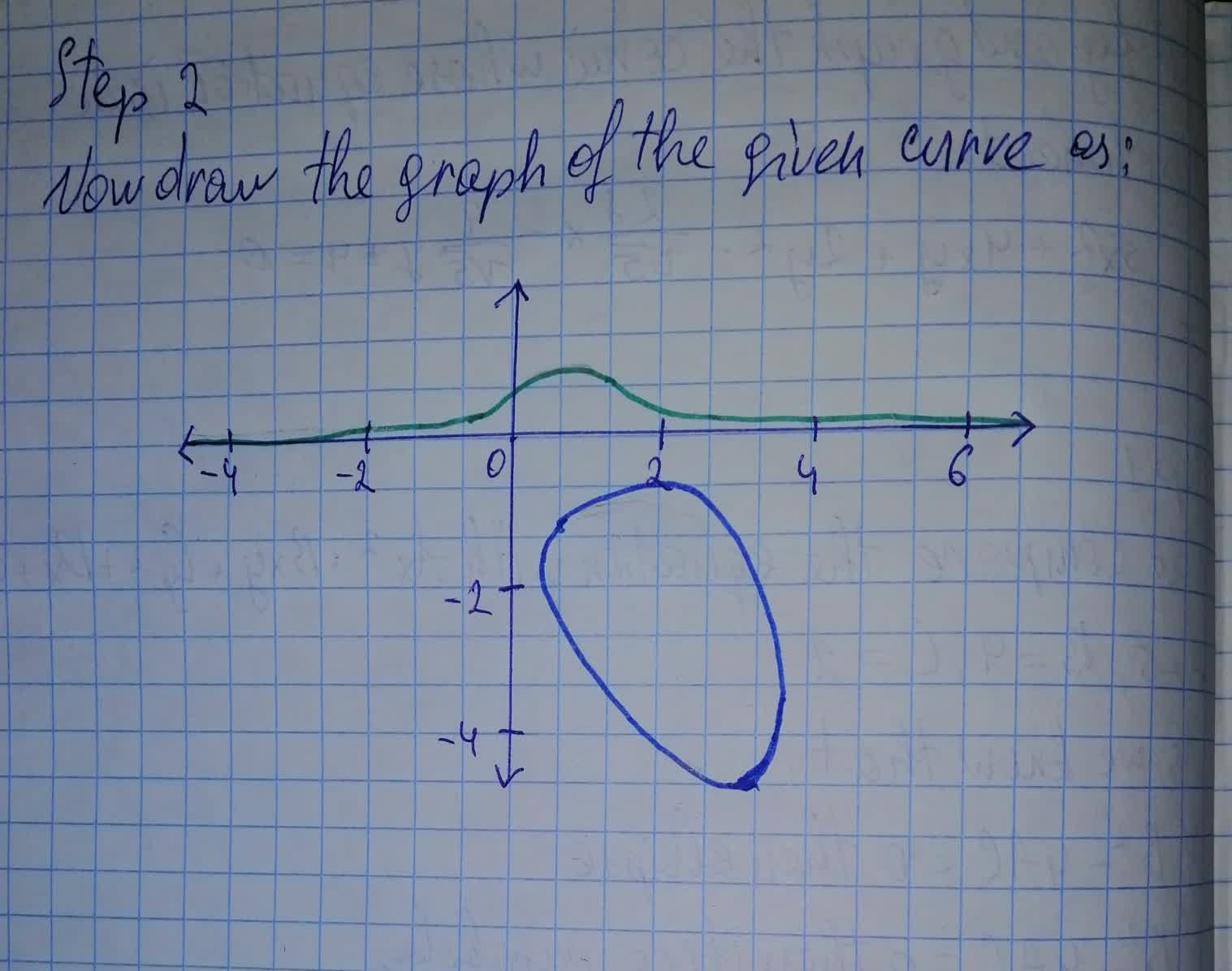Question# Identify and graph the conic whose equation is in attachment.5x^2+4xy+2y^2-frac{28}{\sqrt5}x-\frac{4}{\sqrt5}y+4=0

Conic sections
ANSWEREDIdentify and graph the conic whose equation is in attachment.
$$\displaystyle{5}{x}^{{2}}+{4}{x}{y}+{2}{y}^{{2}}-{\frac{{{28}}}{{\sqrt{{5}}}}}{x}-{\frac{{{4}}}{{\sqrt{{5}}}}}{y}+{4}={0}$$